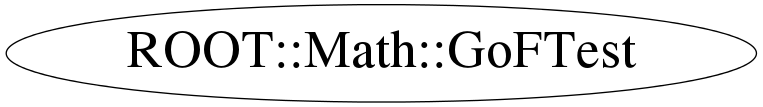# class ROOT::Math::GoFTest

## Function Members (Methods)

public:
 virtual ~GoFTest() Double_t AndersonDarling2SamplesTest(const Char_t* option = "p") const void AndersonDarling2SamplesTest(Double_t& pvalue, Double_t& testStat) const static void AndersonDarling2SamplesTest(const ROOT::Fit::BinData& data1, const ROOT::Fit::BinData& data2, Double_t& pvalue, Double_t& testStat) Double_t AndersonDarlingTest(const Char_t* option = "p") const void AndersonDarlingTest(Double_t& pvalue, Double_t& testStat) const ROOT::Math::GoFTest GoFTest(UInt_t sampleSize, const Double_t* sample, ROOT::Math::GoFTest::EDistribution dist = kUndefined) ROOT::Math::GoFTest GoFTest(UInt_t sample1Size, const Double_t* sample1, UInt_t sample2Size, const Double_t* sample2) ROOT::Math::GoFTest GoFTest(UInt_t sampleSize, const Double_t* sample, const ROOT::Math::IGenFunction& dist, ROOT::Math::GoFTest::EUserDistribution userDist = kPDF, Double_t xmin = 1, Double_t xmax = 0) Double_t KolmogorovSmirnov2SamplesTest(const Char_t* option = "p") const void KolmogorovSmirnov2SamplesTest(Double_t& pvalue, Double_t& testStat) const Double_t KolmogorovSmirnovTest(const Char_t* option = "p") const void KolmogorovSmirnovTest(Double_t& pvalue, Double_t& testStat) const Double_t operator()(ROOT::Math::GoFTest::ETestType test = kAD, const Char_t* option = "p") const void operator()(ROOT::Math::GoFTest::ETestType test, Double_t& pvalue, Double_t& testStat) const static Double_t PValueADKSamples(UInt_t nsamples, Double_t A2) void SetDistribution(ROOT::Math::GoFTest::EDistribution dist) void SetUserCDF(const ROOT::Math::IGenFunction& cdf, Double_t xmin = 1, Double_t xmax = 0) void SetUserDistribution(const ROOT::Math::IGenFunction& dist, ROOT::Math::GoFTest::EUserDistribution userDist = kPDF, Double_t xmin = 1, Double_t xmax = 0) void SetUserPDF(const ROOT::Math::IGenFunction& pdf, Double_t xmin = 1, Double_t xmax = 0)
private:
 Double_t ExponentialCDF(Double_t x) const Double_t GaussianCDF(Double_t x) const static Double_t GetSigmaN(const vector& ns, UInt_t N) ROOT::Math::GoFTest GoFTest() ROOT::Math::GoFTest GoFTest(ROOT::Math::GoFTest& gof) void Instantiate(const Double_t* sample, UInt_t sampleSize) static Double_t InterpolatePValues(int nsamples, Double_t A2) Double_t LogNormalCDF(Double_t x) const void LogSample() ROOT::Math::GoFTest operator=(ROOT::Math::GoFTest& gof) Double_t PValueAD1Sample(Double_t A2) const void SetCDF() void SetDistributionFunction(const ROOT::Math::IGenFunction& cdf, Bool_t isPDF, Double_t xmin, Double_t xmax) void SetParameters() void SetSamples(vector samples, const vector samplesSizes)

## Data Members

public:
 enum EDistribution { kUndefined kUserDefined kGaussian kLogNormal kExponential }; enum EUserDistribution { kCDF kPDF }; enum ETestType { kAD kAD2s kKS kKS2s };
private:
 auto_ptr fCDF vector fCombinedSamples ROOT::Math::GoFTest::EDistribution fDist Double_t fMean vector > fSamples Double_t fSigma Bool_t fTestSampleFromH0

## Class Charts## Function documentation

GoFTest(UInt_t sample1Size, const Double_t* sample1, UInt_t sample2Size, const Double_t* sample2)
```Constructor for using only with 2-samples tests
```
GoFTest(UInt_t sampleSize, const Double_t* sample, ROOT::Math::GoFTest::EDistribution dist = kUndefined)
```Constructor for using only with 1-sample tests with a specified distribution
```
GoFTest(UInt_t sampleSize, const Double_t* sample, const ROOT::Math::IGenFunction& dist, ROOT::Math::GoFTest::EUserDistribution userDist = kPDF, Double_t xmin = 1, Double_t xmax = 0)
```Templated constructor for using only with 1-sample tests with a user specified distribution
```
Instantiate(const Double_t* sample, UInt_t sampleSize)
GoFTest(UInt_t sampleSize, const Double_t* sample, const ROOT::Math::IGenFunction& dist, ROOT::Math::GoFTest::EUserDistribution userDist = kPDF, Double_t xmin = 1, Double_t xmax = 0)
```Specialization using IGenFunction interface
```
SetUserDistribution(const ROOT::Math::IGenFunction& dist, ROOT::Math::GoFTest::EUserDistribution userDist = kPDF, Double_t xmin = 1, Double_t xmax = 0)
SetDistributionFunction(const ROOT::Math::IGenFunction& cdf, Bool_t isPDF, Double_t xmin, Double_t xmax)
void SetUserPDF(const ROOT::Math::IGenFunction& pdf, Double_t xmin = 1, Double_t xmax = 0)
```Sets the user input distribution as a probability density function for 1-sample tests
```
void SetUserCDF(const ROOT::Math::IGenFunction& cdf, Double_t xmin = 1, Double_t xmax = 0)
``` Sets the user input distribution as a cumulative distribution function for 1-sample tests
The CDF must return zero

```
void SetDistribution(ROOT::Math::GoFTest::EDistribution dist)
```Sets the distribution for the predefined distribution types
```
virtual ~GoFTest()
Double_t AndersonDarling2SamplesTest(const Char_t* option = "p") const
```  The Anderson-Darling K-Sample Test algorithm is described and taken from
http://www.itl.nist.gov/div898/software/dataplot/refman1/auxillar/andeksam.htm
and described and taken from
(1) Scholz F.W., Stephens M.A. (1987), K-sample Anderson-Darling Tests, Journal of the American Statistical Association, 82, 918–924. (2-samples variant implemented)
*/ void AndersonDarling2SamplesTest(Double_t& pvalue, Double_t& testStat) const;
```
Double_t AndersonDarlingTest(const Char_t* option = "p") const
```  The Anderson-Darling 1-Sample Test algorithm for a specific distribution is described at
http://www.itl.nist.gov/div898/software/dataplot/refman1/auxillar/andedarl.htm
and described and taken from (2)
Marsaglia J.C.W., Marsaglia G. (2004), Evaluating the Anderson-Darling Distribution, Journal of Statistical Software, Volume 09, Issue i02.
and described and taken from (3)
Lewis P.A.W. (1961), The Annals of Mathematical Statistics, Distribution of the Anderson-Darling Statistic, Volume 32, Number 4, 1118-1124.
*/ void AndersonDarlingTest(Double_t& pvalue, Double_t& testStat) const;
```
Double_t KolmogorovSmirnov2SamplesTest(const Char_t* option = "p") const
```  The Kolmogorov-Smirnov 2-Samples Test algorithm is described at
http://www.itl.nist.gov/div898/software/dataplot/refman1/auxillar/ks2samp.htm
and described and taken from
http://root.cern.ch/root/html/TMath.html#TMath:KolmogorovTest
*/ void KolmogorovSmirnov2SamplesTest(Double_t& pvalue, Double_t& testStat) const;
```
Double_t KolmogorovSmirnovTest(const Char_t* option = "p") const
```  The Kolmogorov-Smirnov 1-Sample Test algorithm for a specific distribution is described at
http://www.itl.nist.gov/div898/software/dataplot/refman1/auxillar/kstest.htm
and described and taken from (4)
Press W. H., Teukolsky S.A., Vetterling W.T., Flannery B.P. (2007), Numerical Recipes - The Art of Scientific Computing (Third Edition), Cambridge Univerdity Press
*/ void KolmogorovSmirnovTest(Double_t& pvalue, Double_t& testStat) const;
```
void operator()(ROOT::Math::GoFTest::ETestType test, Double_t& pvalue, Double_t& testStat) const
``` The class's unary functions
```
Double_t operator()(ROOT::Math::GoFTest::ETestType test = kAD, const Char_t* option = "p") const
``` Returns default Anderson Darling 1-Sample Test and default p-value; option "t" returns the test statistic value
specific to the test type
```
``` Computation of the K-Sample Anderson-Darling Test's p-value as described in (1)
given a normalized test statistic. The first variant described in the paper is used
```
void AndersonDarling2SamplesTest(const ROOT::Fit::BinData& data1, const ROOT::Fit::BinData& data2, Double_t& pvalue, Double_t& testStat)
``` Compute The 2-Sample Anderson Darling test for binned data
```
GoFTest()
GoFTest operator=(ROOT::Math::GoFTest& gof)
void SetCDF()
Double_t LogNormalCDF(Double_t x) const
Double_t GaussianCDF(Double_t x) const
Double_t ExponentialCDF(Double_t x) const
Double_t GetSigmaN(const vector<UInt_t>& ns, UInt_t N)
Double_t InterpolatePValues(int nsamples, Double_t A2)